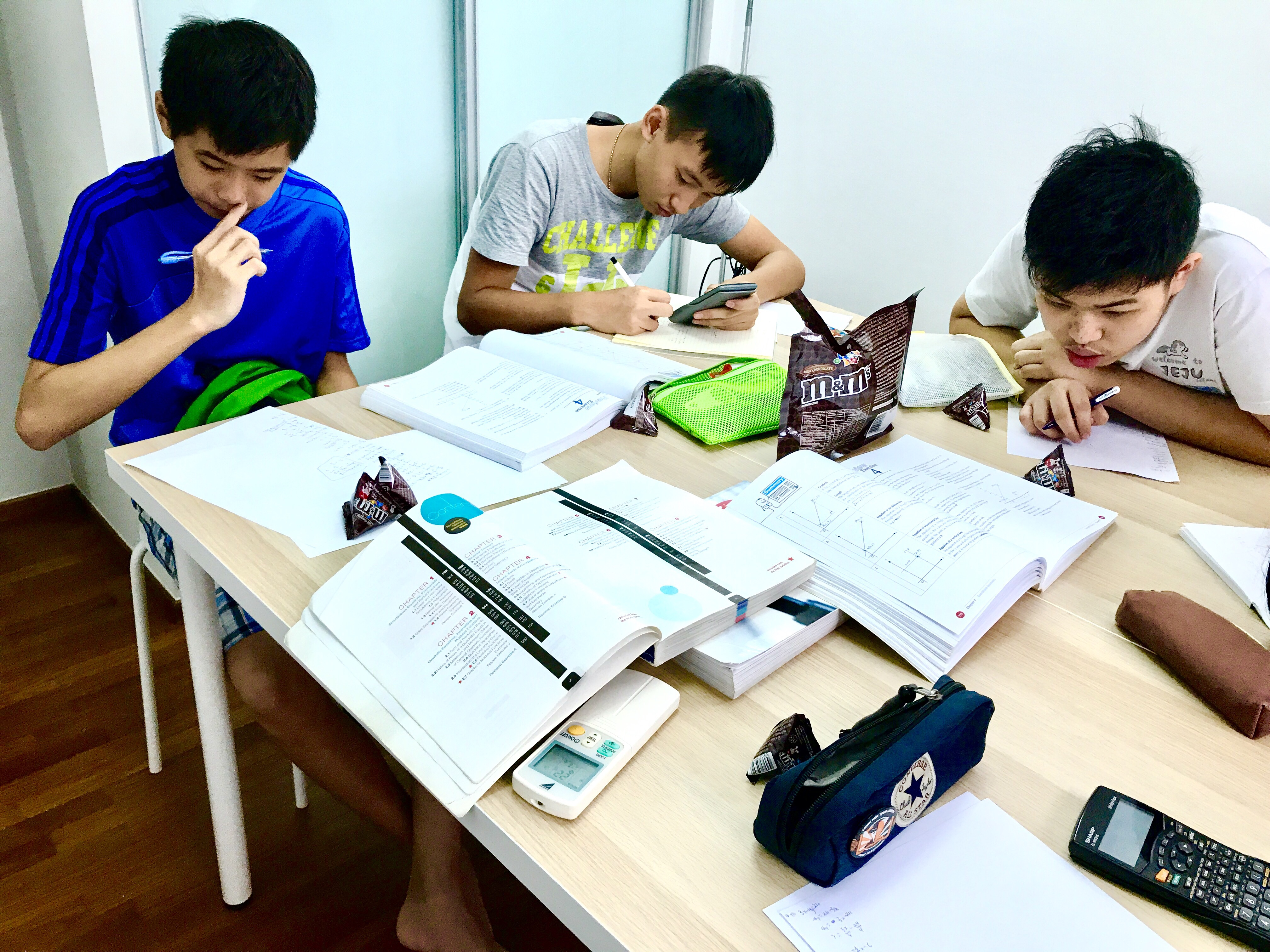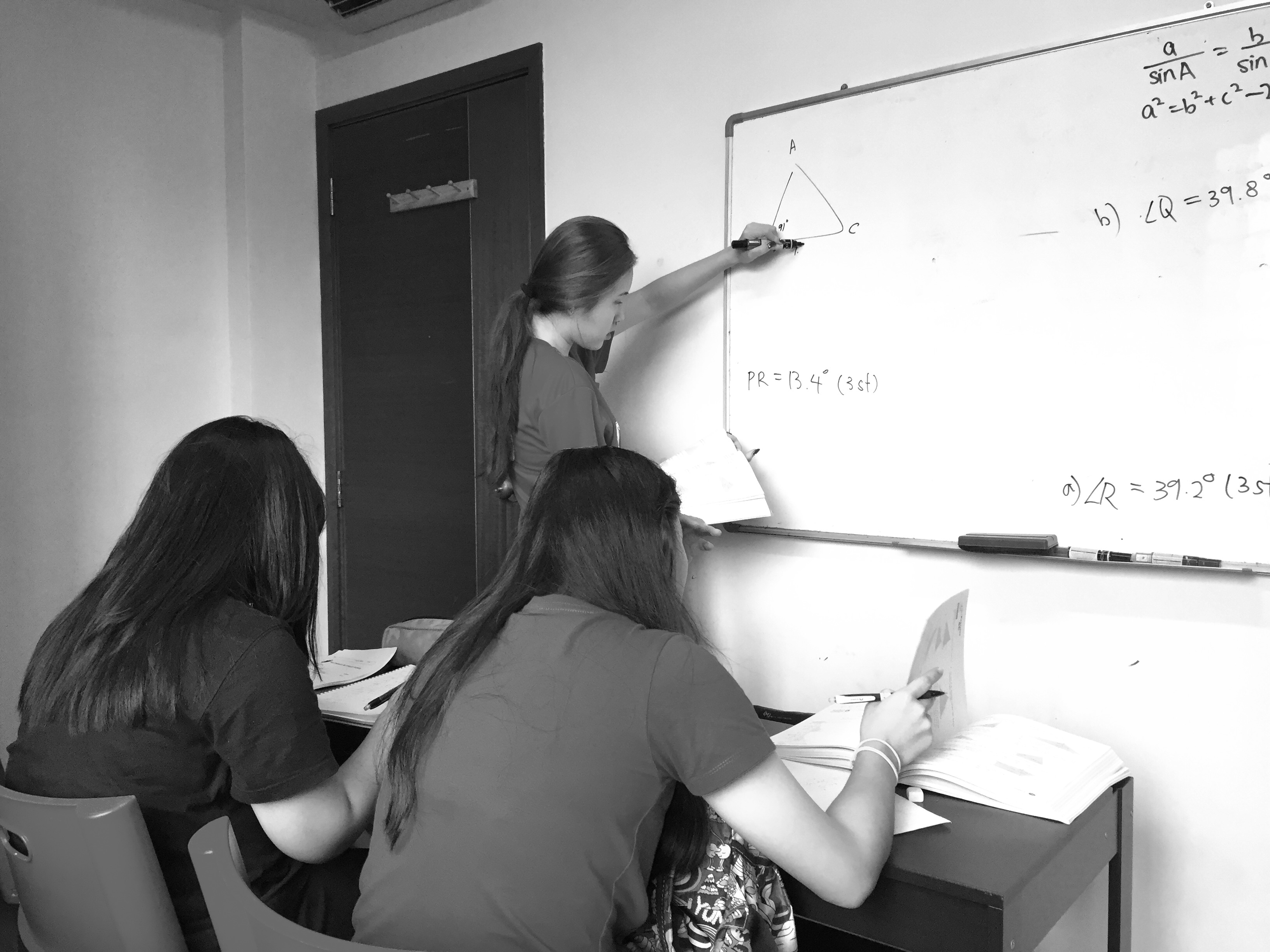# Singapore Sec 1 E Maths Tuition

Sec 1 E Maths Tuition Singapore. E Maths for Secondary 1 (S1) following GCE O-levels, IP, IGCSE and SAP Programme Syllabus in Singapore. Best Tutors from ex-RJC, SAJC, TPJC, ACJC uni grads and our previous students have achieved double A1 distinctions for A and E Maths consistently.

Exam preparations and techniques are taught in class and we specialise in getting weaker students to understand E Maths HCF LCM Algebra, Factorisation, Expansion, Equations, and improve tremendously to score A1.

Our Singapore Sec 1 Math course teaches all topics outlined by MOE Singapore Mathematics SEAB GCE O-level E Maths Syllabus. We teach from fundamentals from scratch, and we teach comprehensively to include every trick needed to score a distinction in the examination. We also provide all materials needed including past year exam papers.

#### Sec 1 E Maths Topics

• Primes, HCF, LCM
• Integers, Rational, Real Numbers
• Approximation and Estimation
• Basic Algebra and Algebraic Manipulation
• Linear Equations and Simple Inequalities
• Functions and Linear Graphs
• Number Patterns
• Percentage Rate Ratio and Speed